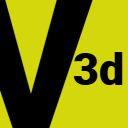# sourcefes2vector3d::V3d.fan

```**
** Title: V3d
** Author: Fernando Raya
** Created: 12 Dec 16
**

**
** 'V3d' represents a three dimension vector with 'Float' coordinates
** 'x', 'y' and 'z'.
**
** 'V3d' has the following operators overloaded:
**
** - Addition: 'a + b' given 'a' and 'b' of 'V3d' type.
** - Substraction: 'a - b' given 'a' and 'b' of 'V3d' type.
** - Negation: '-a' given 'a' of 'V3d' type.
** - Scalar multiplication: 'a * n' given 'n' of type 'Float' or 'Int'
** - Scalar division: 'a / n' given 'n' of type 'Float'
**
** References
** **********
**
** - `https://www.codeproject.com/articles/17425/a-vector-type-for-c`
** - `http://nrich.maths.org/2390`
** - `http://nrich.maths.org/2393`
** - `http://introcs.cs.princeton.edu/java/34nbody/`
**
@Serializable
@Js
const class V3d
{
**
** 'x' coordinate
**
const Float x

**
** 'y' coordinate
**
const Float y

**
** 'z' coordinate
**
const Float z

**
** Vector with all components initialized to 0.0f
**
const static V3d zero := V3d(0.0f, 0.0f, 0.0f)

**
** Make a new vector
**
new make(Float x, Float y, Float z)
{
this.x = x
this.y = y
this.z = z
}

**
** Add a vector to 'this'. Shortcut is 'this + that'
**
@Operator
This plus(V3d that)
{
V3d(x + that.x, y + that.y, z + that.z)
}

**
** Negation.
** Shortcut is '-a'
**
@Operator
This negate()
{
V3d(-x, -y, -z)
}

**
** Substract that from 'this'.
** Shortcut is 'this - that'
**
@Operator
This minus(V3d that)
{
V3d(x - that.x, y - that.y, z - that.z)
}

**
** Multiplication by a scalar.
** Shortcut is 'this * 3.0f'
**
@Operator
This multFloat(Float n)
{
V3d(x * n, y * n, z * n)
}

**
** Multiplication by a scalar.
** Equivalent to 'multFloat(n.toFloat)'.
** Shortcut is 'this * 3'
**
@Operator
This multInt(Int n)
{
this * n.toFloat
}

**
** Division by a scalar.
** Shortcut is 'this / 3.0f'
**
@Operator
This divFloat(Float n)
{
V3d(x / n, y / n, z / n)
}

**
** Dot product
**
Float dot(V3d that)
{
(x * that.x) + (y * that.y) + (z * that.z)
}

**
** Sum of the squared components 'x', 'y' and 'z'
**
Float squared()
{
this.dot(this)
}

**
** Length of the vector obtained from Pythagoras's theorem.
**
Float magnitude()
{
squared.sqrt
}

**
** Cross product
**
V3d cross(V3d that)
{
V3d
(
y * that.z - z * that.y,
z * that.x - x * that.z,
x * that.y - y * that.x
)
}

**
** Absolute value, alias for 'magnitude'
**
Float abs()
{
magnitude
}

**
** Size, alias for 'magnitude'
**
Float size()
{
magnitude
}

**
** Is the unit vector?
** 'true' if 'magnitude' is 1.0f, 'false' otherwise.
**
Bool isUnit()
{
magnitude == 1.0f
}

**
** Distance of 'this' to 'that'
**
Float distance(V3d that)
{
(this - that).magnitude
}

**
** 'true' if is instance of 'V3d' and the coordinates are equal
**
override Bool equals(Obj? that)
{
if (that isnot V3d) return false
other := (V3d) that
return x == other.x && y == other.y && z == other.z
}

**
** Comparison of vectors is based in their magnitudes
**
override Int compare(Obj that)
{
other := (V3d) that
return magnitude.compare(other.magnitude)
}

override Int hash()
{
// TODO: Just guessing
x.hash.xor(y.hash).xor(z.hash)
}

**
** Show the vector as a 'Str' '(x,y,z)'
**
override Str toStr()
{
"(\${x},\${y},\${z})"
}
}
```